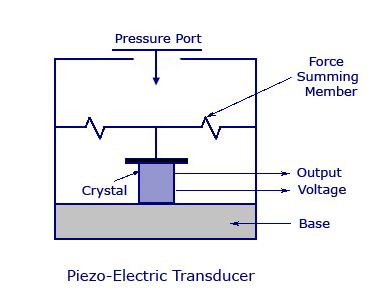# Piezoelectric transducer

The piezoelectric transducers are used to convert mechanical energy into electrical energy. Asymmetrical crystalline materials such as quartz, Rochelle salt and barium titanate produce an emf when they are placed under stress. This property is used in piezoelectric transducer where crystal is placed between a solid base and force summing membrane. This force summing membrane is used to distribute the applied pressure equally on the surface of crystal, so that accurate result can be obtained.When external force is applied to transducer through its pressure port an emf is produced across the crystal. The produced emf is proportional to the magnitude of applied pressure. As the device does not require any external supply and therefore it is self generating. The output voltage is given by,

E=Q/Cp

Where,

Cp = Shunt capacitance.

Q = Generated charge.

The piezoelectric effect is direction sensitive. A tensile force produces a voltage of opposite polarity. The materials used in piezoelectric transducer are Rochelle salt, quartz, barium titanate etc.